# DFA String Examples

Design a DFA over w ∈ {a,b}* such that number of a = 2 and there is no restriction over length of b.
We will explain this intuitive approach
Step 1 Make smallest string DFA:
Means for number of a’s = 2 , crate a DFA then in next step we will take care of b’s.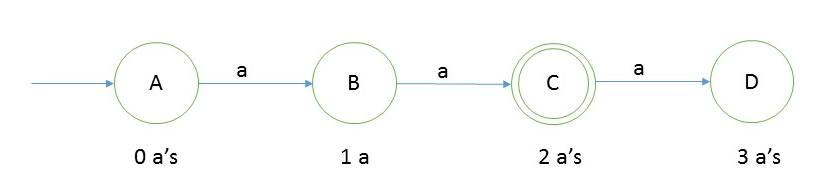Step 2
Now we will take care of b’s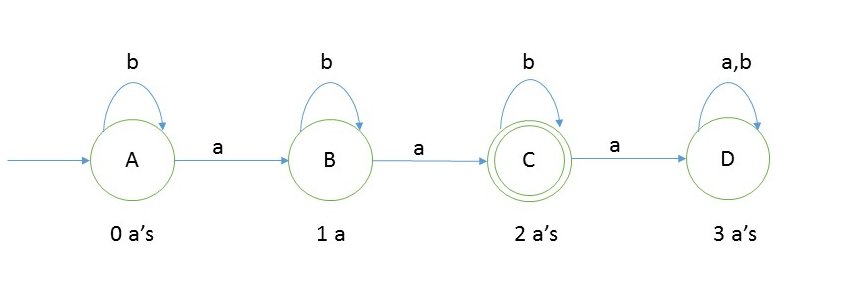You can test any string which has number of a’s = 2, will be accepted in the above.
Design a DFA in which number of a’s in the string are ≥ 2

Like above we will create DFA taking care of number of a’s ≥ 2, and input alphabet is a, b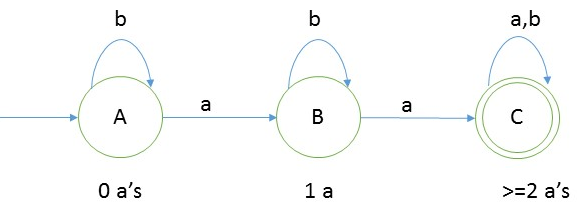So in this way we will take care of the language, L = {aa, aab, baba, bbaa, aabb, …….}
It is pretty clear that language is infinite.

Design a DFA in which number of a’s in the string are ≤ 2

Like above we will create DFA taking care of number of a’s ≤ 2, and input alphabet is a, b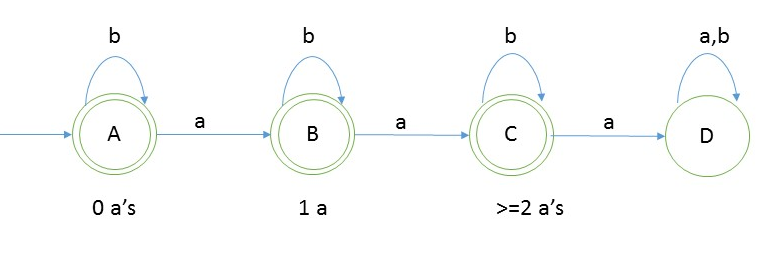So in this way we will take care of the language, L = {∈ b, a, ab, bba, bba, abb, …….}
It is pretty clear that language is infinite.

#### Testing

1. Take a string ‘ababb’ to test whether it is accepted in the above DFA
2. Scan string from left to right
3. first input we get is a and from start state(A) on ‘a’ we go to B
4. second input is b, from state B on b we will go to state B itself.
5. third input is a, from B we go to state C.
6. from state C we go to state B on fourth input symbol C itself
7. from C on fifth symbol b, we will go to state C itself.
8. Now we reached end of string and we are standing on final state C.
So string ababb is accepted in the language.

#### Negative Testing

1. Take a string ‘ababba’ to test whether it is accepted in the above DFA
2. Scan string from left to right
3. first input we get is a and from start state(A) on ‘a’ we go to B
4. second input is b, from state B on b we will go to state B itself.
5. third input is a, from B we go to state C.
6. from state C we go to state B on fourth input symbol C itself
7. from C on fifth symbol b, we will go to state C itself.
8. from C on sixth symbol a, we will go to state D
9. Now we reached end of string and we are not standing on final state C.
So we can say that string ababba is not accepted in the language.

Design a DFA in which number of a’s are divisible by 2. And input alphabet is {a,b}
Answer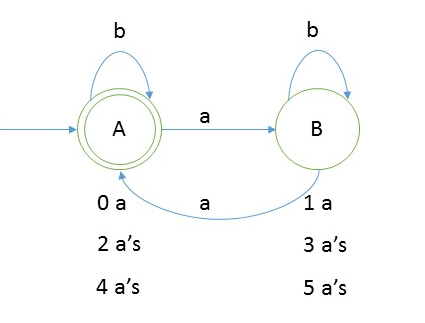And if you check any string in the language, L = {b, aab,aa, aaaab, aabbbbbbb, …… }, this DFA will accept it. And if the string do not follow the property will not be accepted.

In the similar ground we can create DFA in which number of a’s are divisible by 2 with remainder 1.
Means -> #a mod 2 =1